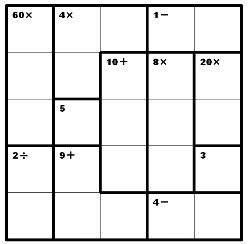# Flash Fun: KENKEN, the new puzzle craze

By web gangsta | Published:

Now that you’ve finally figured out how to play SUDOKU puzzles, isn’t it about time to take your numbers grid skills to the next level?  Sure it is –
try KENKEN!kenken

A kenken puzzle is relatively straightforward.  Just like in sudoku, you have to fill in numbers in rows and columns without duplicates.   But there’s a catch….The major difference is that instead of filling in numbers from 0-9 in each row and column like you would in sudoku, in kenken you are filling in numbers from 1-x, where “x” is the size of the puzzle.  In our example here, we would fill in numbers from 1-5 in every row and every column.   A 4×4 square would have us filling in numbers from1-4 in every row and every column.

The difference is that the rows and columns are broken out into highlighted cages that contain a mathematical problem that needs to be solved with your inputted numbers.  The numbers you enter, when combined (in any order) must match the number in that cage’s section using the mathematical symbol indicated.  So a 2-square cage labelled “3+” must contain a 1 and a 2, as 1+2=3.  Similarly, a 2-square cage labelled “11+” must contain 5+6.  In a 5×5 grid, a 3-square cage containing “10x” can only contain 1*2*5.

Solving strategy:

• First, the easy part: if there are any single boxes (cages), fill in those numbers with their displayed digits.
• Are there any cages that have very high or very low totals for their number of squares?  Chances are, there will be just one possible set of numbers that will fit correctly.
• Even though you can’t repeat numbers in a row or a column, a cage can span different areas of the grid. This will allow you to repeat numbers in a cage (so you could have 4+4+1=9 in a single 3-square cage).
• The order the numbers appear in a cage do not matter.
• Just like in sudoku, each row and column only contain the numbers once.  If your math fails you, then try your sudoko skills to reason out the rest.